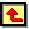# Appendix II. SEM and LRS Data

This appendix presents scanning electron micrographs and laser Raman spectra relevant to the results presented within Chapters 5-7.  The appendix is split into three sections which contain data for films grown using various (a) Tsub using 50%CH4/50%CO2 gas mixtures, (b) H2S additions to 1%CH4/H2 gas mixtures and (c) H2S additions to 51%CH4/49%CO2 gas mixtures

## (a). 50%CH4/50%CO2 Gas Mixtures

All the following data is for diamond films deposited on n-type Si (100) wafers under the conditions outlined below (See Chapter 5).

 Gas Mixture 50%CH4/50%CO2 Total Flow rate / sccm 80 Applied Microwave Power / W 1000 Chamber Pressure / Torr 40 Substrate Temperature Varies between different films

The sample code, Tsub and growth rate of each film is also given.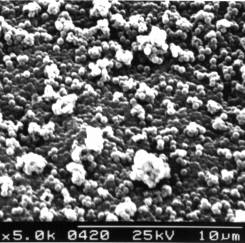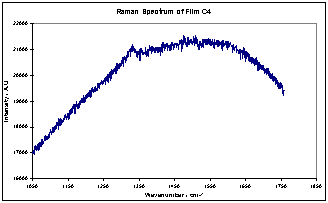(Sample C4, Tsub = 434°C, Growth Rate = 0.075 mm h-1)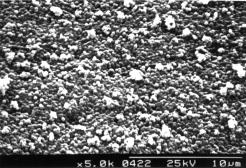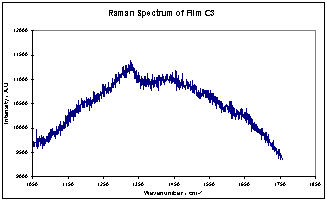(Sample C3, Tsub = 512°C, Growth Rate = 0.101 mm h-1)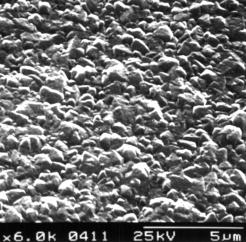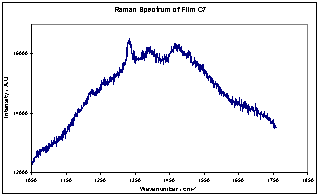(Sample C7, Tsub = 590°C, Growth Rate = 0.171 mm h-1)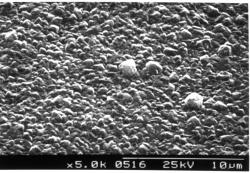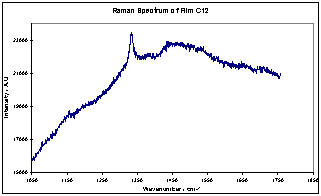(Sample C12, Tsub = 645°C, Growth Rate = 0.213 mm h-1)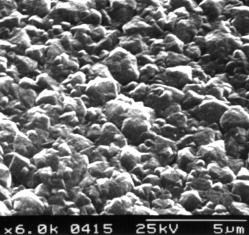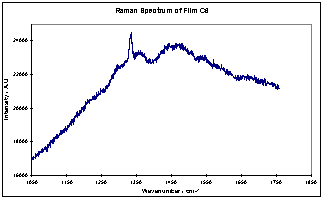(Sample C6, Tsub = 649°C, Growth Rate = 0.181 mm h-1)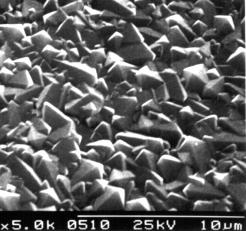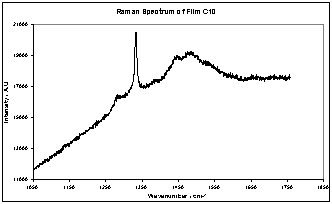(Sample C10, Tsub = 843°C, Growth Rate = 0.398 mm h-1)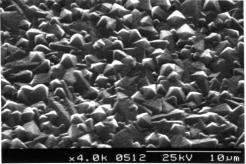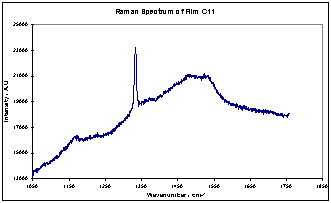(Sample C11, Tsub = 863°C, Growth Rate = 0.427 mm h-1)

## (b). H2S/1%CH4/H2 Gas Mixtures

All the following data is for diamond films deposited on Si (100) wafers under the conditions outlined below (see Chapter 6).  It should be noted that for the sake of clarity, curve fits are not shown for these Raman spectra.

 Gas Mixture H2S/1%CH4/H2 Total Flow rate / sccm 200 H2S Addition Varies between films Applied Microwave Power / W 1000 Chamber Pressure / Torr 40 Substrate Temperature / °C 900

The sample code, substrate type and gas phase H2S addition for each film is given.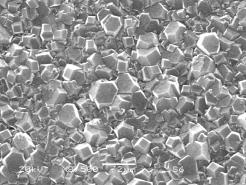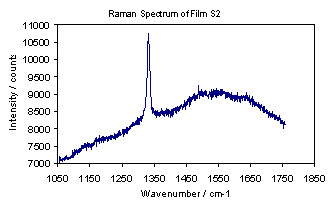(Sample S2, n-type Si, 50 ppm H2S addition)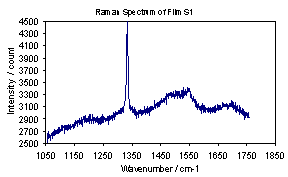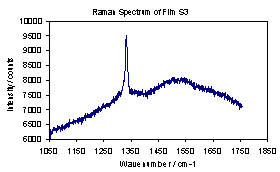(Sample S1, n-type Si, 100 ppm H2S addition/Sample S3, n-type Si,200 ppm H2S addition)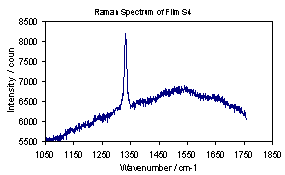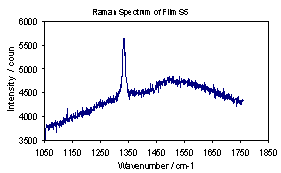(Sample S4, n-type Si, 400 ppm H2S addition/ Sample S5, n-type Si, 500 ppm H2S addition)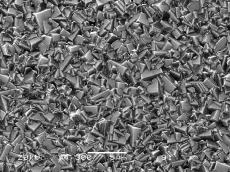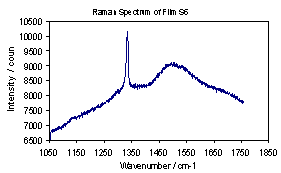(Sample S6, n-type Si, 1000 ppm H2S addition)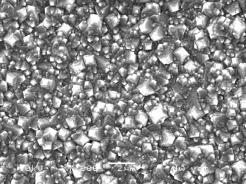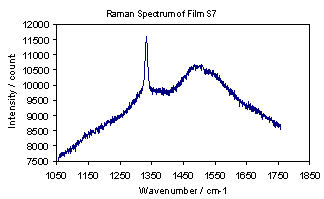(Sample S7, n-type Si, 5000 ppm H2S addition)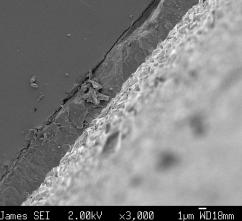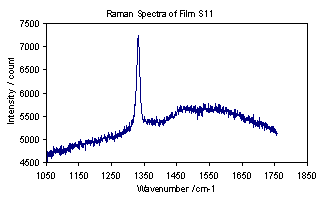(Sample S11, undoped Si, 0 ppm H2S addition, Growth rate = 0.575 mm h-1)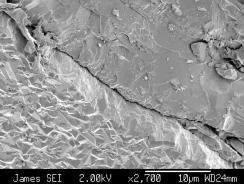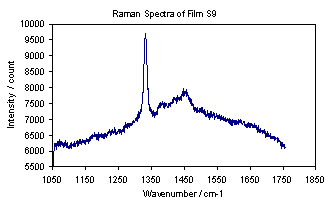(Sample S9, undoped Si, 1000 ppm H2S addition, Growth rate = 0.543 mm h-1)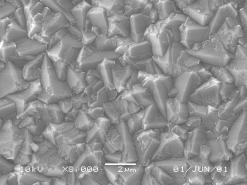(Sample S20, undoped Si, 3000 ppm H2S addition, Growth rate = 0.531 mm h-1)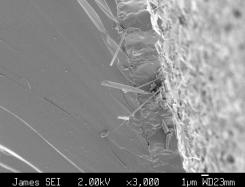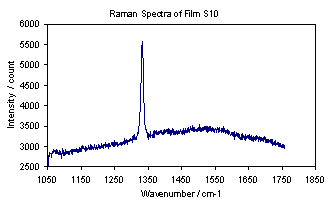(Sample S10, undoped Si, 5000 ppm H2S addition, Growth rate = 0.500 mm h-1)

## (c). H2S/51%CH4/49%CO2 Gas Mixtures

All the following data is for diamond films deposited on undoped Si (100) wafers under the conditions outlined below (see Chapter 7).

 Gas Mixture H2S/51%CH4/49%CO2 Total Flow rate / sccm 100 H2S Addition Varies between films Applied Microwave Power / W 1000 Chamber Pressure / Torr 40 Substrate Temperature / °C 900

The sample code, Tsub, gas phase H2S addition and growth rate for each film is given.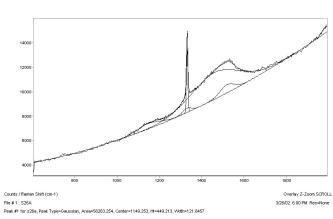(Sample S26, Tsub 620°C, 0 ppm H2S addition, Growth Rate = 0.239 mm h-1)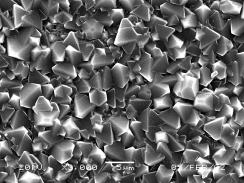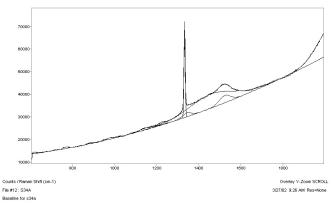(Sample S34, Tsub 620°C, 100 ppm H2S addition, Growth Rate = 0.230 mm h-1)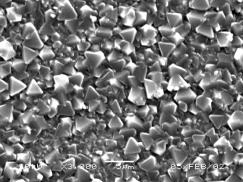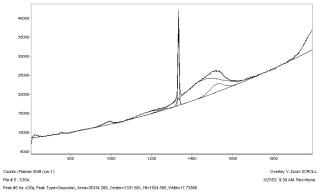(Sample S35, Tsub 620°C, 200 ppm H2S addition, Growth Rate = 0.257 mm h-1)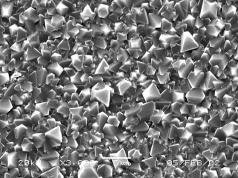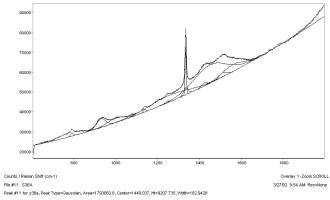(Sample S36, Tsub 620°C, 400 ppm H2S addition, Growth Rate = 0.178 mm h-1)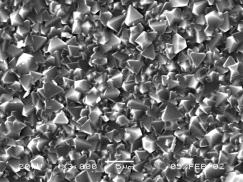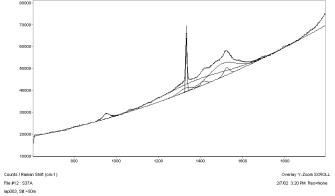(Sample S37, Tsub 620°C, 600 ppm H2S addition, Growth Rate = 0.232 mm h-1)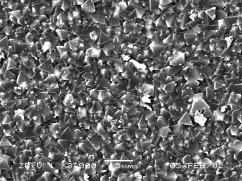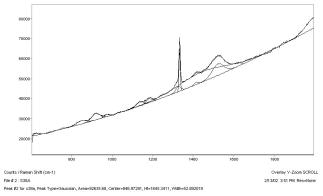(Sample S38, Tsub 620°C, 800 ppm H2S addition, Growth Rate = 0.156 mm h-1)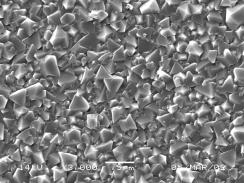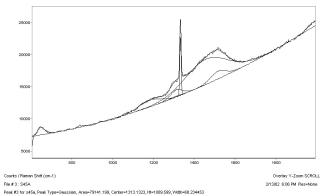(Sample S45, Tsub 620°C, 2000 ppm H2S addition, Growth Rate = 0.177 mm h-1)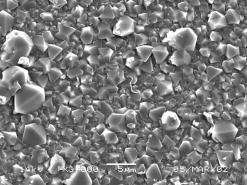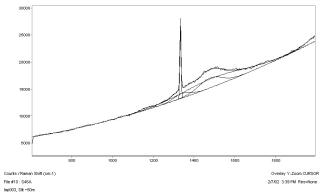(Sample S46, Tsub 620°C, 3500 ppm H2S addition, Growth Rate = 0.132 mm h-1)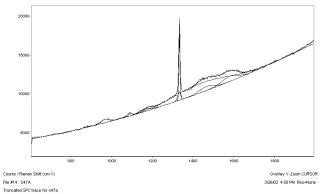(Sample S47, Tsub 620°C, 5000 ppm H2S addition, Growth Rate = 0.103 mm h-1)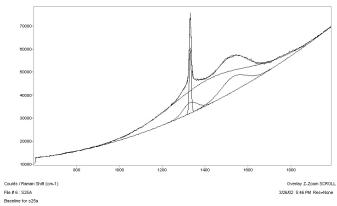(Sample S25, Tsub 900°C, 0 ppm H2S addition, Growth Rate = 3.00 mm h-1)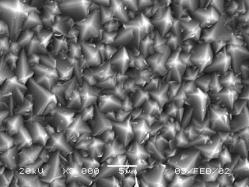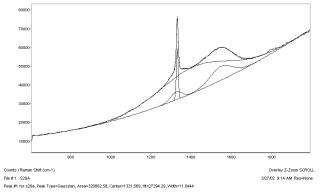(Sample S29, Tsub 900°C, 100 ppm H2S addition, Growth Rate = 2.10 mm h-1)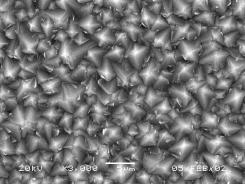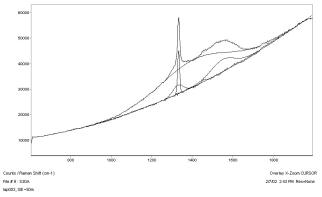(Sample S30, Tsub 900°C, 200 ppm H2S addition, Growth Rate = 2.43 mm h-1)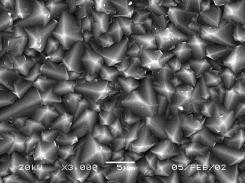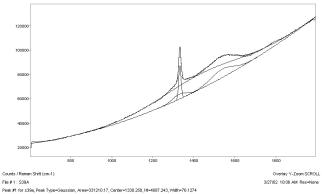(Sample S39, Tsub 900°C, 400 ppm H2S addition, Growth Rate = 2.04 mm h-1)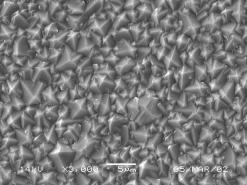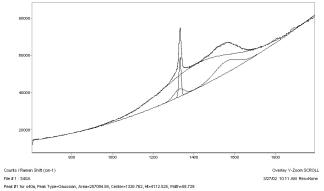(Sample S40, Tsub 900°C, 600 ppm H2S addition, Growth Rate = 1.39 mm h-1)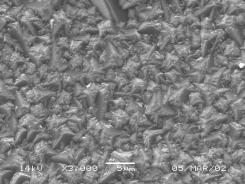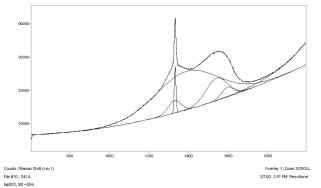(Sample S41, Tsub 900°C, 800 ppm H2S addition, Growth Rate = 1.89 mm h-1)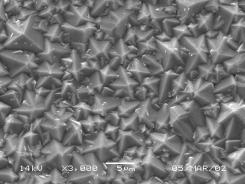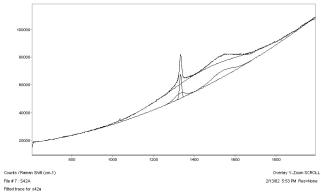(Sample S42, Tsub 900°C, 2000 ppm H2S addition, Growth Rate = 1.48 mm h-1)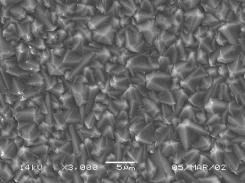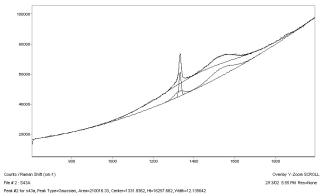(Sample S43, Tsub 900°C, 3500 ppm H2S addition, Growth Rate = 1.56 mm h-1)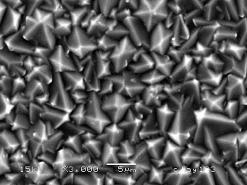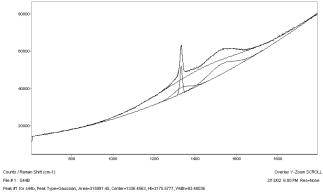(Sample S44, Tsub 900°C, 5000 ppm H2S addition, Growth Rate = 1.28 mm h-1)